# 通用技术 性能测试与 “正则表达式”

JoyMao · 2022年05月26日 · 最后由 JoyMao 回复于 2022年06月02日 · 3412 次阅读

### 说明

（特别说明，我的正则水平不高，“指数型正则”、“嵌套型正则 “是根据现象给的叫法，勿较真）

### 邮箱格式校验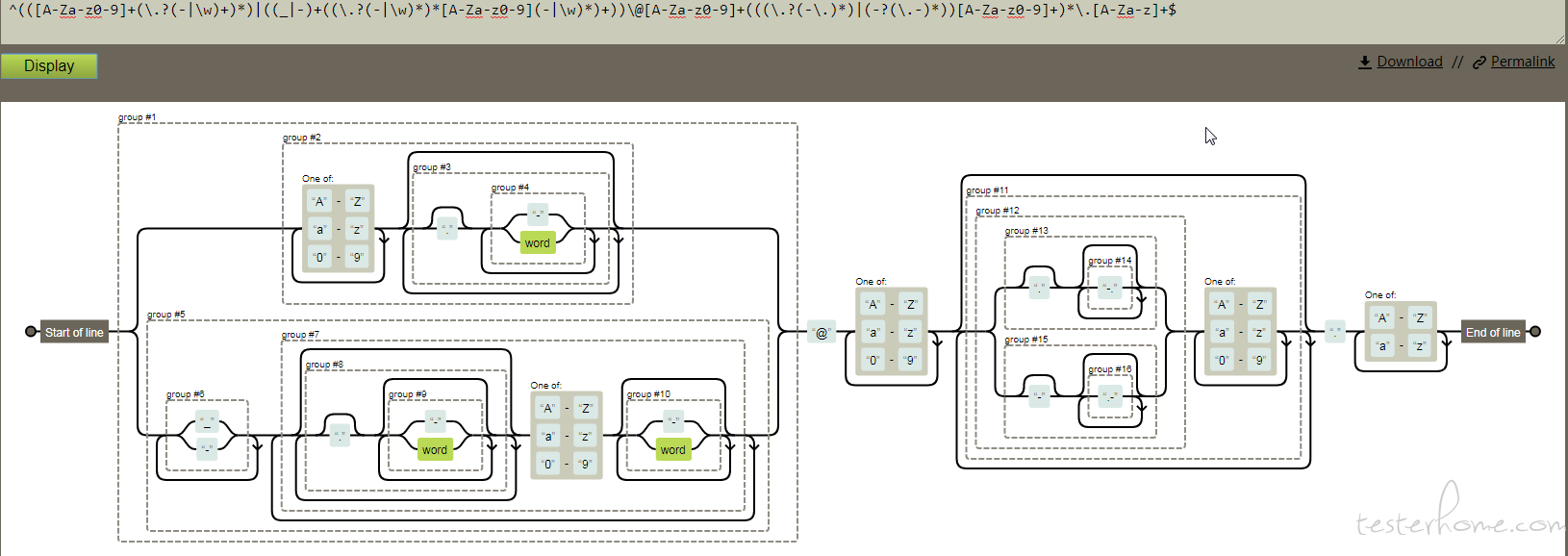``````分析：
(\.?(-|\w)+)*)[A-Za-z0-9]这个是明显的指数型正则特征

``````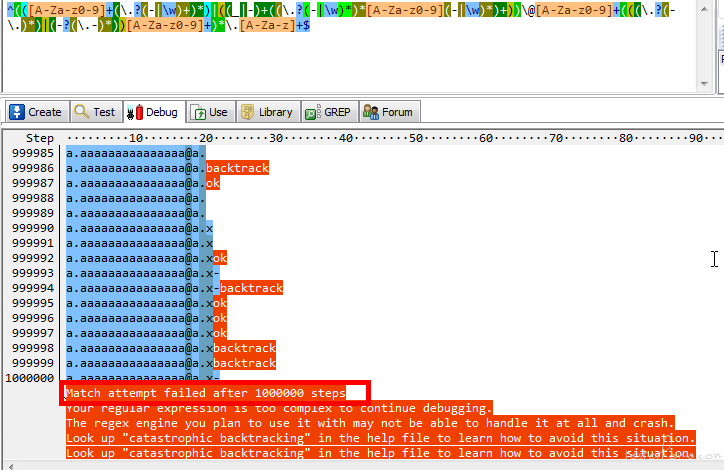``````补充：

``````

### sql 注入检查正则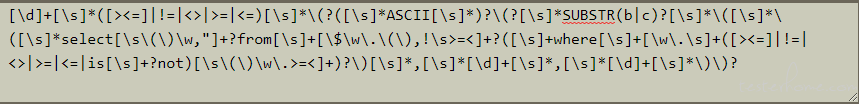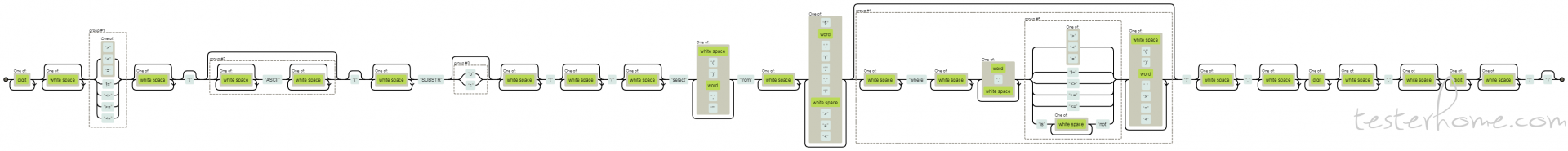``````分析：
from后面涵盖匹配项包含了where及其后续的内容，即where处即可认为是“数据库表名”又可匹配后面的where处内容。
``````

``````1>((substr((select from A where 1= where 1= where 1= where 1= where 1= where 1= where 1= where 1= where 1= where 1= where 1= where 1= where 1= where 1= where 1= where 1= where 1= where 1= where 1= where 1=/),1,1))
``````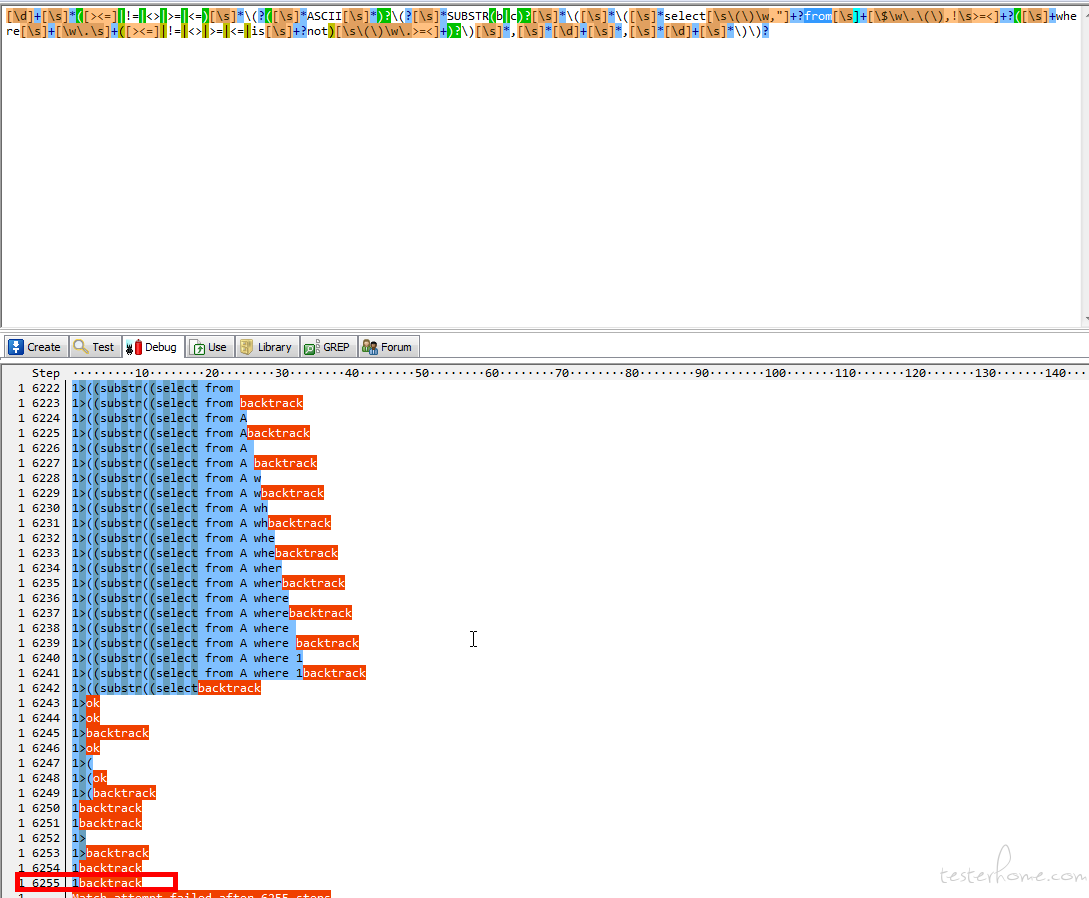tips: 正则可视化网站:https://regexper.com/

#2 · 2022年06月02日 Author

https://zhuanlan.zhihu.com/p/456349063

#1 · 2022年06月02日 Author